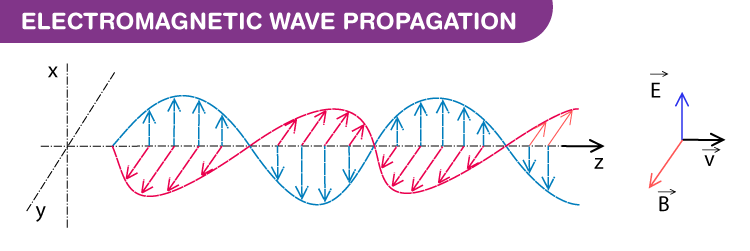# Wave Theory of Light

## Introduction

• In the late 17th century, scientists were embroiled in a debate about the fundamental nature of light – whether it was a wave or a particle.
• Sir Isaac Newton strongly advocated the particle nature of light.
• But, the Dutch physicist Christiaan Huygens believed that light was made up of waves vibrating up and down perpendicular to the direction of the wave propagation and therefore formulated a way of visualizing wave propagation.
• This became known as ‘Huygens’ Principle‘.

The wave theory of light proposed by Christian Huygens has stood the test of time, and today, it is considered the backbones of optics. Here, in the article, let us discuss the wave theory of light in detail.

## History Of The Wave Theory Of Light

Light always piqued the curiosity of thinkers and scientists. But it wasn’t until the late 17th century that scientists began comprehending light’s properties. Sir Isaac Newton proposed that light was made of tiny particles known as photons, while Christian Huygens believed that light was made of waves propagating perpendicular to the direction of its movement.

In 1678, Huygens proposed that every point that a luminous disturbance meets turns into a source of the spherical wave itself. The sum of the secondary waves, which are the result of the disturbance, determines what form the new wave will take. This theory of light is known as the ‘Huygens’ Principle’.

Using the above-stated principle, Huygens successfully derives the laws of reflection and refraction of light. He was also successful in explaining the linear and spherical propagation of light using this theory. However, he wasn’t able to explain the diffraction effects of light. Later, in 1803, the experiment conducted by Thomas Young on the interference of light proved the Huygens wave theory of light to be correct. Later in 1815, Fresnel provided mathematical equations for Young’s experiment.

Max Planck proposed that light is made of finite packets of energy known as a light quantum and depends on light’s frequency and velocity. Later, in 1905, Einstein proposed that light possessed particle and wave characteristics. He suggested that light is made of small particles called photons. Quantum mechanics gave proof of the dual nature of light.

## Light Wave TheoryMost of the time, light behaves as a wave, categorized as one of the electromagnetic waves because it is made of electric and magnetic fields. Electromagnetic fields perpendicularly oscillate to the direction of wave travel and are perpendicular to each other. As a result of which, they are known as transverse waves. A few characteristics of light are as follows:

• While dealing with light waves, we deal with the sine waveform. The period of the waveform is one full 0 to 360-degree sweep.
• Light waves have two important characteristics known as wavelength and frequency.
• The distance between the peaks of the wave is known as the wavelength. In the case of a light wave, the wavelengths are in the order of nanometers.
• Frequency is the number of waves crossing past a point in a second.
• The relationship between wavelength and frequency is given by the equation:
$$\begin{array}{l}f=\frac{1}{T}\end{array}$$
• The speed of light in a vacuum is a universal constant which is 3 x 108 m/s.
• As proposed by Einstein, light is made of tiny packets of energy known as photons. The formula devised by Planck determines the energy of a photon, and it also shows that the energy is directly proportional to the frequency of the light.

E = hf, where h is the Planck’s constant 6.63 × 10-34 Joule-Second

Although neither Huygens nor Newton realized it, they had tapped the two critical aspects of one of the most crucial questions in physics – the fundamental nature of light. Stay tuned to BYJU’S to learn more about capacitors, inductors, and more.

## Watch the video and understand how constructive and destructive interferences are formed.## Frequently Asked Questions – FAQs

Q1

### What is a wave?

In physics, a wave is a moving, dynamic disturbance of matter or energy in an organised and periodic way.
Q2

### What is light?

Light is defined as the electromagnetic wave within the section of the electromagnetic spectrum that is visible to the human eye.
Q3

### Who proposed the wave theory of light?

Christian Huygens proposed the wave theory of light.
Q4

### What did Max Planck propose about the nature of light?

Max Planck proposed that light is made of finite packets of energy known as a light quantum, and it depends on the frequency and velocity of light.
Q5

### What was the conclusion of Albert Einstein about the nature of light?

In 1905, Einstein proposed that light possessed the characteristics of both particle and wave.
Test your knowledge on Wave Theory Of Light